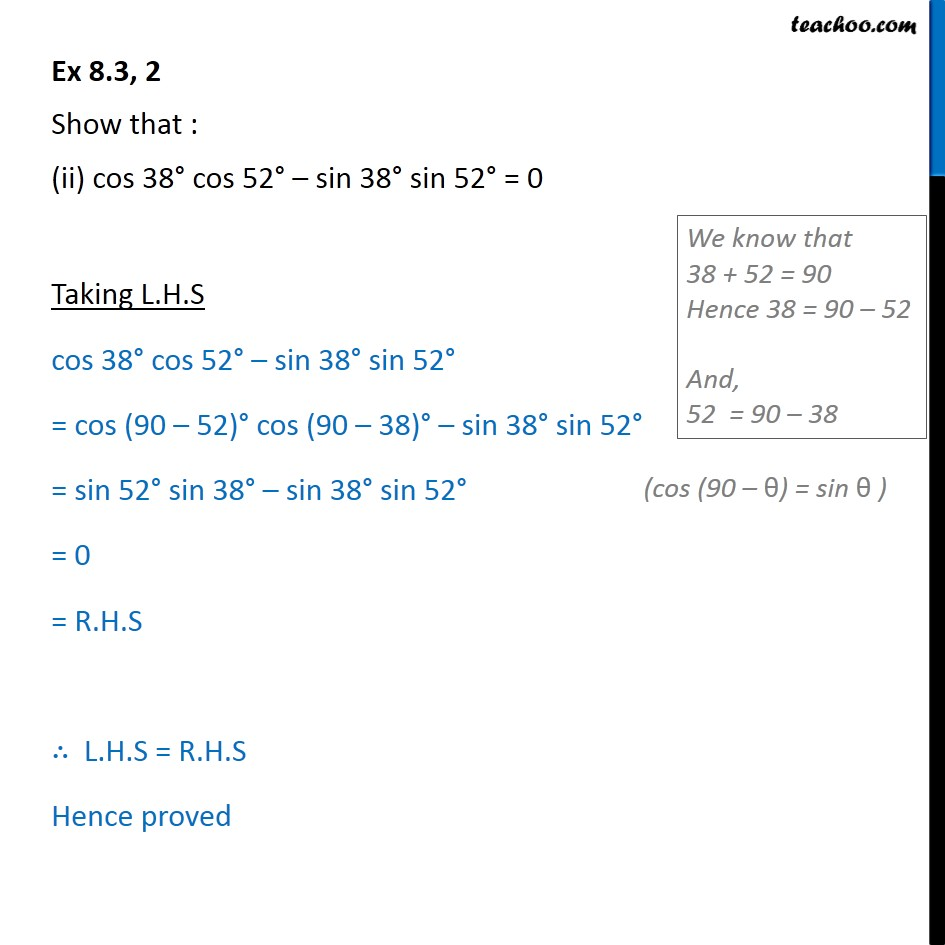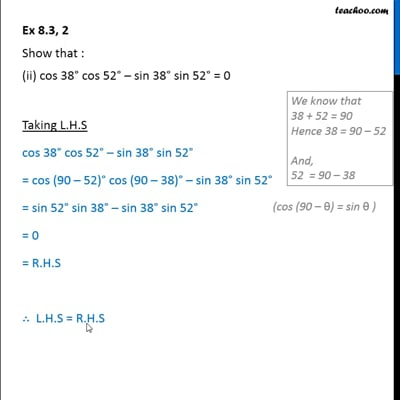Ex 8.3

Chapter 8 Class 10 Introduction to Trignometry
Serial order wiseThis video is only available for Teachoo black users

Maths Crash Course - Live lectures + all videos + Real time Doubt solving!

### Transcript

Ex 8.3, 2 Show that : (ii) cos 38 cos 52 sin 38 sin 52 = 0 Taking L.H.S cos 38 cos 52 sin 38 sin 52 = cos (90 52) cos (90 38) sin 38 sin 52 = sin 52 sin 38 sin 38 sin 52 = 0 = R.H.S L.H.S = R.H.S Hence proved